# Streamgraph in R

Marc Taylor

## Sample data

The `plotStream` function of the `sinkr` package needs a vector of `x` axis values and a matrix `y` containing the data series as columns corresponding to `x`.

In this block of code we are generating a vector with 500 values and a matrix with 30 columns (series) and 500 rows, one for each observation.

The data series have been simulated making use of smoothed splines.

``````set.seed(1)
m <- 500
n <- 30
x <- seq(m)
y <- matrix(0, nrow = m, ncol = n)
colnames(y) <- seq(n)
for(i in seq(ncol(y))) {
mu <- runif(1, min = 0.25 * m, max = 0.75 * m)
SD <- runif(1, min = 5, max = 20)
TMP <- rnorm(1000, mean = mu, sd = SD)
HIST <- hist(TMP, breaks = c(0, x), plot = FALSE)
fit <- smooth.spline(HIST\$counts ~ HIST\$mids)
y[, i] <- fit\$y
}
y <- replace(y, y < 0.01, 0)``````

## Stream visualization plot

Basic stream plot

In order to create a stream plot you can pass the numeric vector and the matrix to the `plotStream` function. Note that a random wiggle is applied to the plot, so if you want to create always the same illustration set a seed.

``````# install.packages("remotes")
# remotes::install_github("marchtaylor/sinkr")
library(sinkr)

# Streamgraph
set.seed(1)
plotStream(x, y,
xlim = c(100, 400),
ylim = c(-125, 125))``````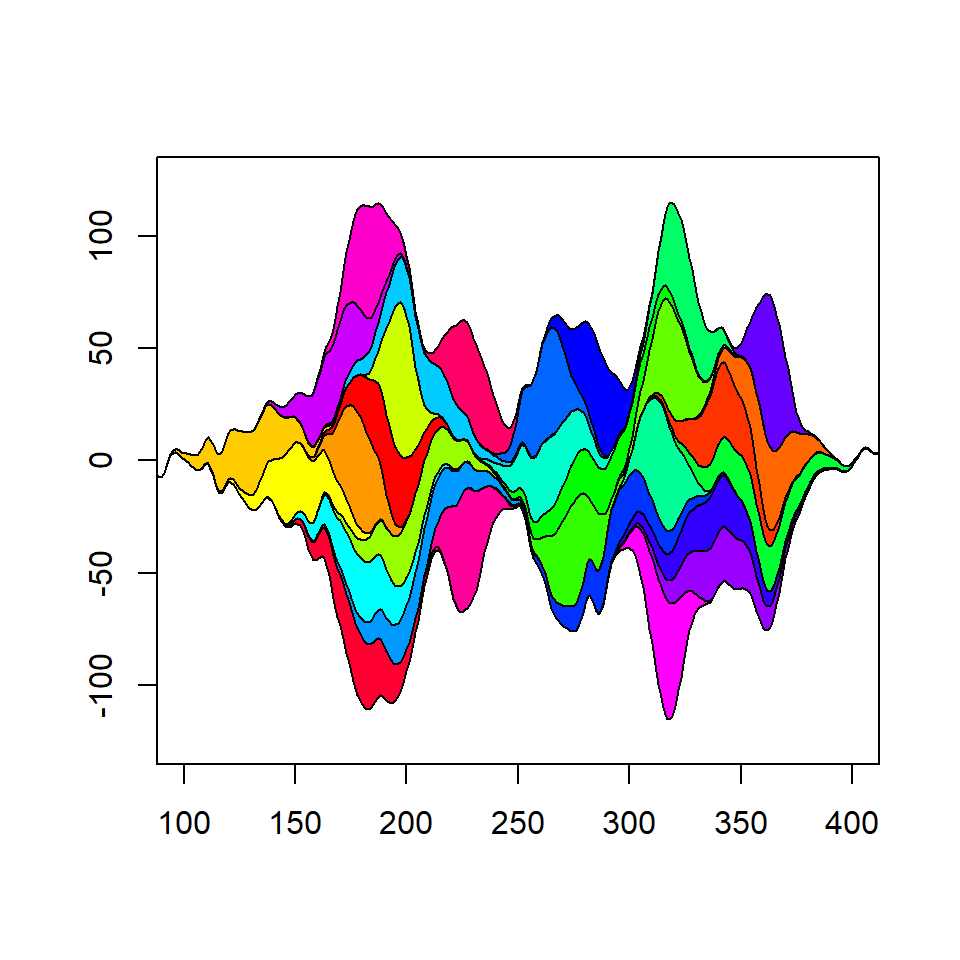Wiggle

The amount of wiggle applied can be customized with the `frac.rand` and `spar` arguments. The first controls the fraction of the overall data stream used to define the range of the wiggle while the latter controls the smoothness of baseline.

``````# install.packages("remotes")
# remotes::install_github("marchtaylor/sinkr")
library(sinkr)

# Streamgraph
set.seed(1)
plotStream(x, y,
xlim = c(100, 400),
ylim = c(-125, 125),
frac.rand = 0.2,
spar = 0.5)``````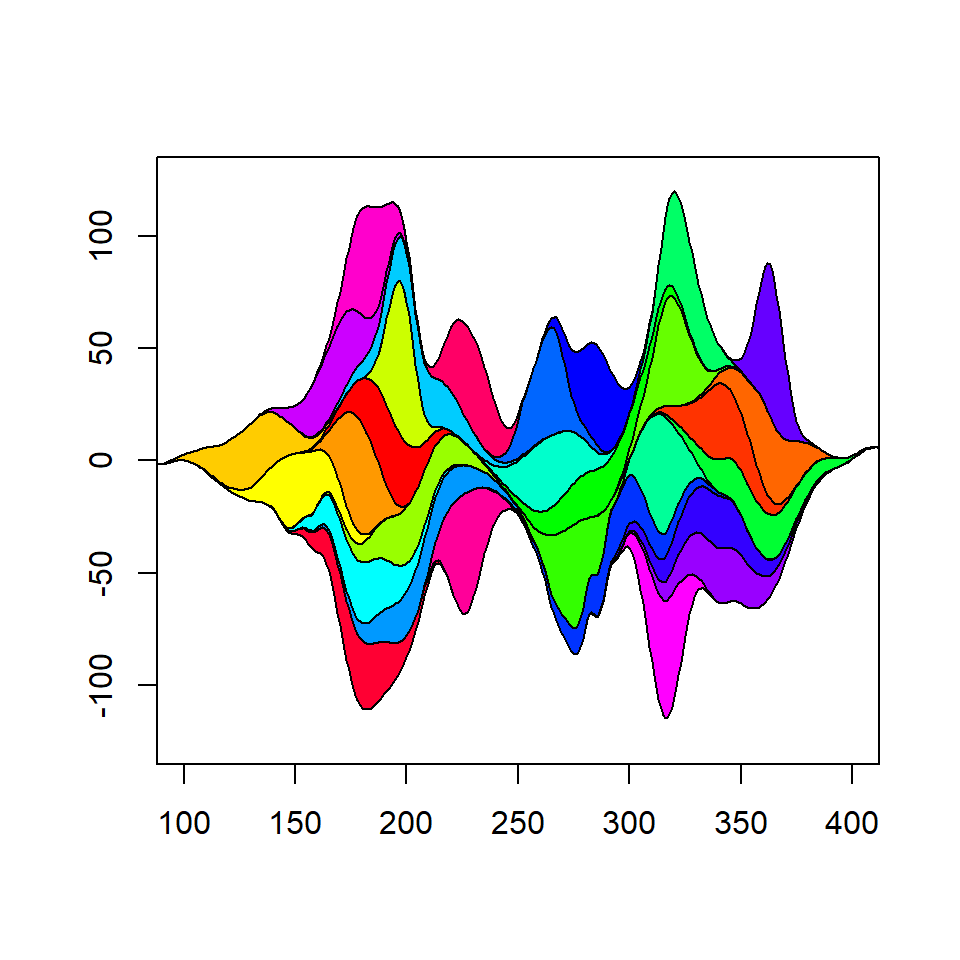Color palette

The default color palette is the rainbow palette. However, you can customize it with the `col` argument. In the example below we are generating a palette with `hcl.colors` of 30 colors (the number of columns of `y`).

``````# install.packages("remotes")
# remotes::install_github("marchtaylor/sinkr")
library(sinkr)

# Colors
cols <- hcl.colors(30, "BluYl")

# Streamgraph
set.seed(1)
plotStream(x, y,
xlim = c(100, 400),
ylim = c(-125, 125),
col = cols)``````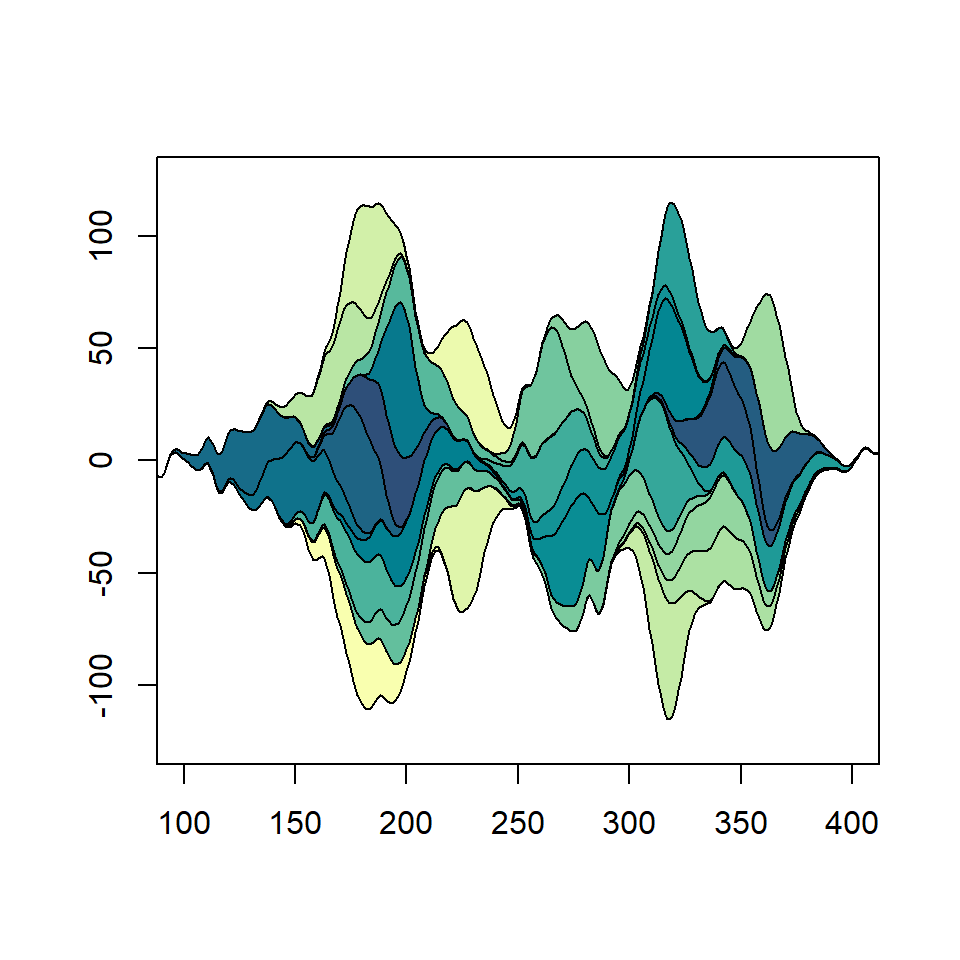Border customization

The color, line width and line type of the polygons used to create the plot can be customized with the `border`, `lwd` and `lty` arguments, respectively.

``````# install.packages("remotes")
# remotes::install_github("marchtaylor/sinkr")
library(sinkr)

# Streamgraph
set.seed(1)
plotStream(x, y, xlim = c(100, 400),
ylim = c(-125, 125),
col = hcl.colors(30, "Blues 3"),
border = "white", lwd = 1, lty = 1)``````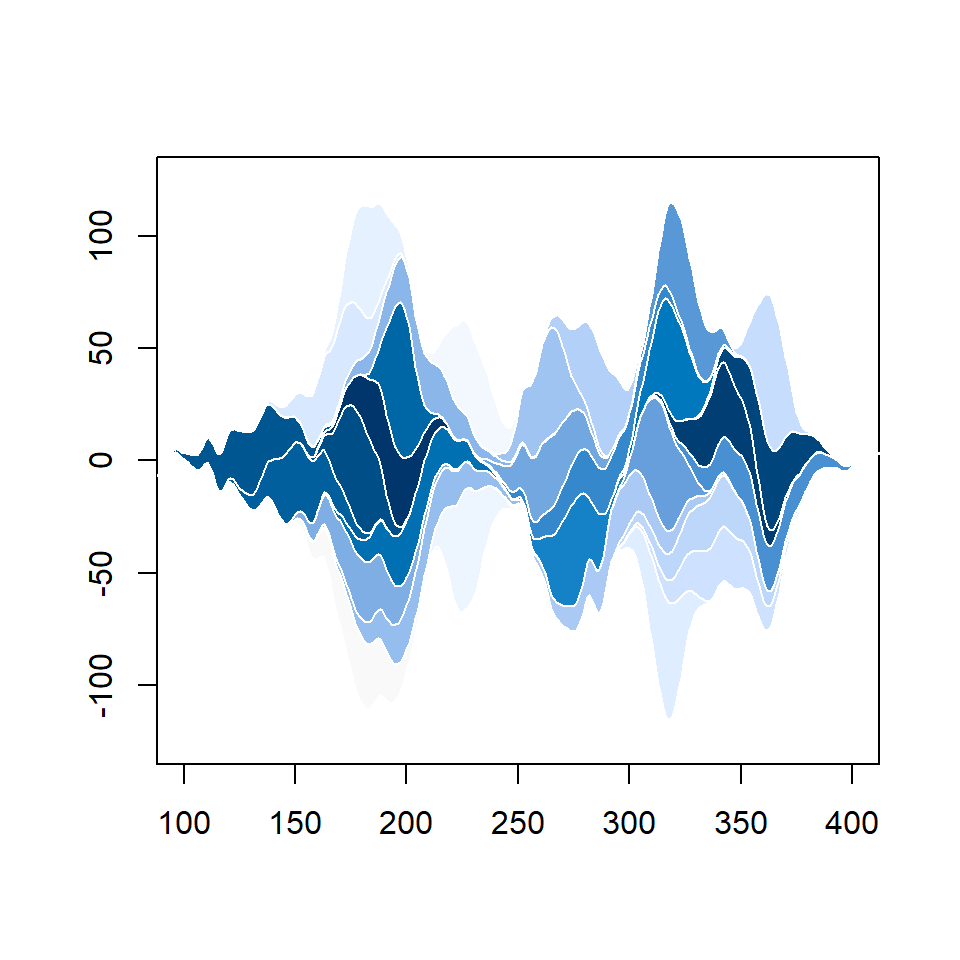## Order methods

The function provides three different ordering plotting methods with the `order.method` argument. The default is `"as.is"`, but you can also set `"max"` or `"first"`. Type `?plotStream` for further details.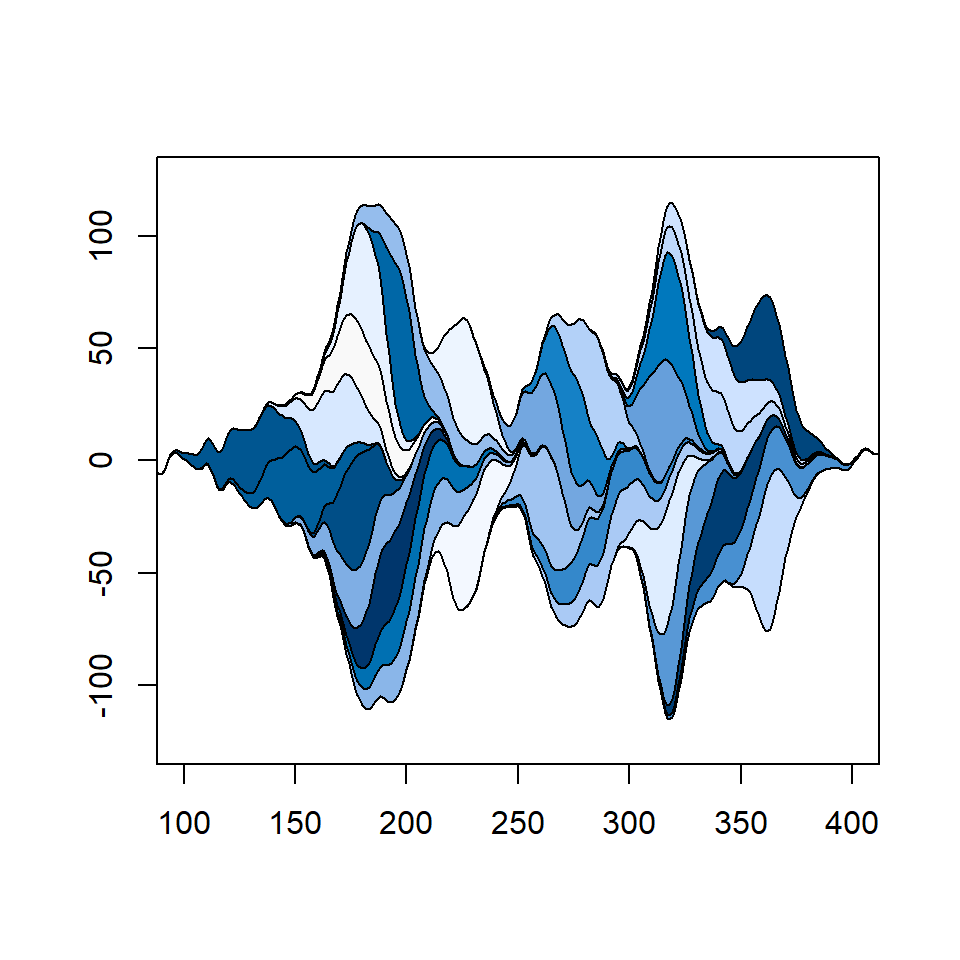“max” order method

``````# install.packages("remotes")
# remotes::install_github("marchtaylor/sinkr")
library(sinkr)

# Streamgraph
set.seed(1)
plotStream(x, y, xlim = c(100, 400),
ylim = c(-125, 125),
col = hcl.colors(30, "Blues 3"),
order.method = "max")``````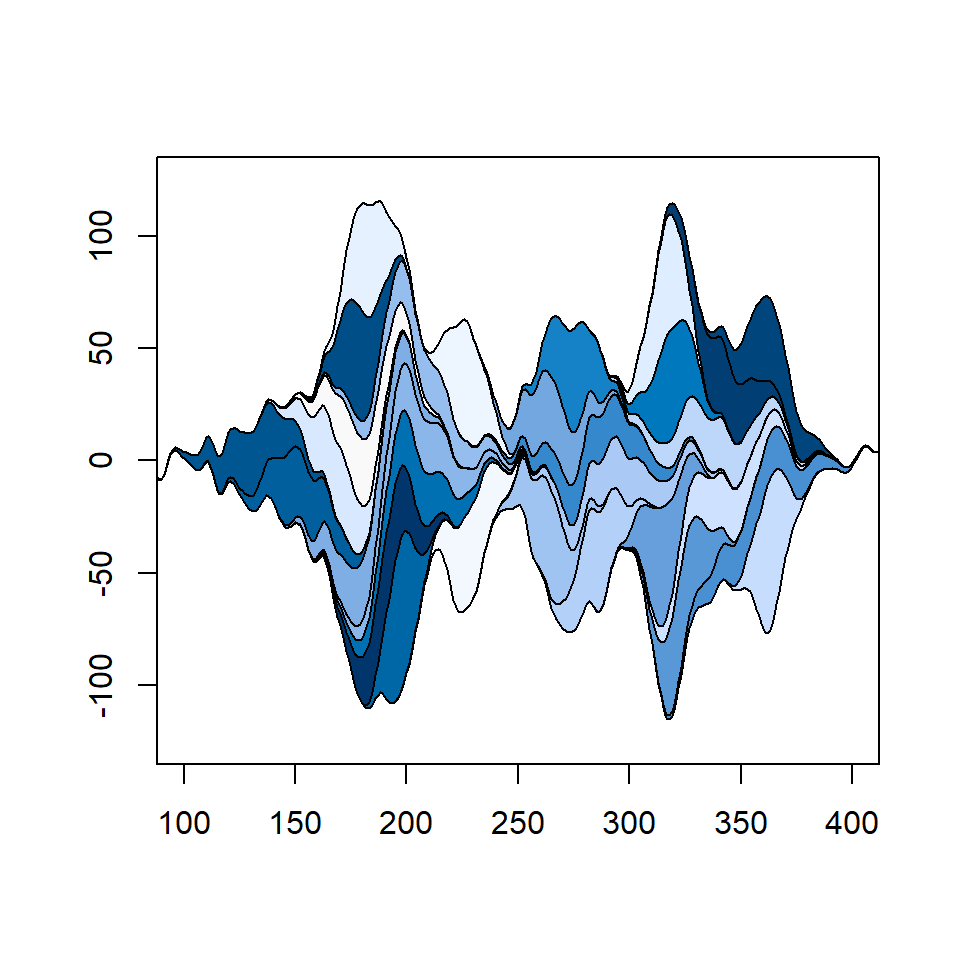“first” order method

``````# install.packages("remotes")
# remotes::install_github("marchtaylor/sinkr")
library(sinkr)

# Streamgraph
set.seed(1)
plotStream(x, y, xlim = c(100, 400),
ylim = c(-125, 125),
col = hcl.colors(30, "Blues 3"),
order.method = "first")``````